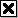# Sallen–Key topology

(Redirected from Sallen Key filter)

The Sallen–Key topology is an electronic filter topology used to implement second-order active filters that is particularly valued for its simplicity . It is a degenerate form of a voltage-controlled voltage-source (VCVS) filter topology. A VCVS filter uses a super-unity-gain voltage amplifier with practically infinite input impedance and zero output impedance to implement a 2-pole (12 dB/octave) low-pass, high-pass, or bandpass response. The super-unity-gain amplifier allows for very high Q factor and passband gain without the use of inductors. A Sallen–Key filter is a variation on a VCVS filter that uses a unity-gain amplifier (i.e., a pure buffer amplifier with 0 dB gain). It was introduced by R.P. Sallen and E. L. Key of MIT Lincoln Laboratory in 1955.

Because of its high input impedance and easily selectable gain, an operational amplifier in a conventional non-inverting configuration is often used in VCVS implementations.[citation needed] Implementations of Sallen–Key filters often use an operational amplifier configured as a voltage follower; however, emitter or source followers are other common choices for the buffer amplifier.

VCVS filters are relatively resilient to component tolerance, but obtaining high Q factor may require extreme component value spread or high amplifier gain. Higher-order filters can be obtained by cascading two or more stages.

## Generic Sallen–Key topology

The generic unity-gain Sallen–Key filter topology implemented with a unity-gain operational amplifier is shown in Figure 1. The following analysis is based on the assumption that the operational amplifier is ideal.

Because the operational amplifier (OA) is in a negative-feedback configuration, its v+ and v- inputs must match (i.e., v+ = v-). However, the inverting input v- is connected directly to the output vout, and so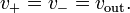$v_+ = v_- = v_{\text{out}}.\,$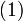$(1)\,$

By Kirchhoff's current law (KCL) applied at the vx node,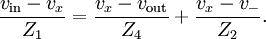$\frac{v_{\text{in}}-v_x}{Z_1}=\frac{v_x-v_{\text{out}}}{Z_4}+\frac{v_x-v_-}{Z_2}.$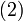$(2)\,$

By combining Equations (1) and (2),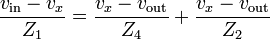$\frac{v_{\text{in}}-v_x}{Z_1}=\frac{v_x-v_{\text{out}}}{Z_4}+\frac{v_x-v_{\text{out}}}{Z_2}$

Applying KCL again at the OA's non-inverting input v+ (= v- = vout) gives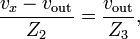$\frac{v_x-v_{\text{out}}}{Z_2}=\frac{v_{\text{out}}}{Z_3},$

which means that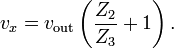$v_x=v_{\text{out}} \left( \frac{Z_2}{Z_3}+1 \right).$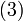$(3)\,$

Combining Equations (2) and (3) gives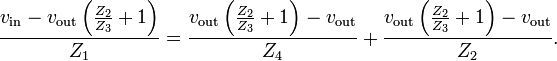$\frac{v_{\text{in}}-v_{\text{out}} \left( \frac{Z_2}{Z_3}+1 \right)}{Z_1}=\frac{v_{\text{out}} \left( \frac{Z_2}{Z_3}+1 \right)-v_{\text{out}}}{Z_4}+\frac{v_{\text{out}} \left( \frac{Z_2}{Z_3}+1 \right)-v_{\text{out}}}{Z_2}.$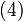$(4)\,$

Rearranging Equation (4) gives the transfer function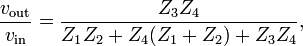$\frac{v_{\text{out}}}{v_{\text{in}}} = \frac{Z_3 Z_4}{Z_1 Z_2 + Z_4(Z_1 + Z_2) + Z_3 Z_4},$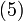$(5)\,$

which typically describes a second-order LTI system.

### Interpretation

If the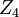$Z_4\,$ component was connected to ground, the filter would be a voltage divider composed of the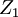$Z_1\,$ and$Z_4\,$ components cascaded with another voltage divider composed of the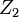$Z_2\,$ and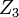$Z_3\,$ components. The buffer bootstraps the "bottom" of the$Z_4\,$ component to the output of the filter, which will improve upon the simple two divider case. This interpretation is the reason why Sallen–Key filters are often drawn with the operational amplifier's non-inverting input below the inverting input, thus emphasizing the similarity between the output and ground.

### Example applications

By choosing different passive components (e.g., resistors and capacitors) for$Z_1\,$,$Z_2\,$,$Z_3\,$, and$Z_4\,$, the filter can be made with low-pass, bandpass, and high-pass characteristics. In the examples below, recall that a resistor with resistance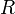$R\,$ has impedance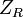$Z_R\,$ of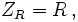$Z_R = R\,,$

and a capacitor with capacitance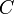$C\,$ has impedance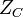$Z_C\,$ of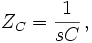$Z_C = \frac{1}{s C}\,,$

where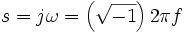$s = j \omega = \left(\sqrt{-1}\right) 2 \pi f\,$ and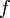$f\,$ is a frequency of a pure sine wave input. That is, a capacitor's impedance is frequency dependent and a resistor's impedance is not.

#### Example: Low-pass filter

An example of a unity-gain low-pass configuration is shown in Figure 2.

An operational amplifier is used as the buffer here, although an emitter follower is also effective. This circuit is equivalent to the generic case above with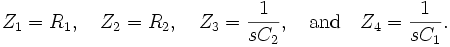$Z_1 = R_1, \quad Z_2 = R_2, \quad Z_3 = \frac{1}{s C_2}, \quad \text{and} \quad Z_4 = \frac{1}{s C_1}.\,$

The transfer function for this second-order unity-gain low-pass filter is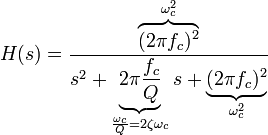$H(s) = \frac{ \overbrace{ ( 2 \pi f_c )^2 }^{ \omega_c^2 } }{ s^2 + \underbrace{ 2 \pi \frac{ f_c }{Q} }_{\frac{\omega_c}{Q} = 2 \zeta \omega_c }s + \underbrace{( 2 \pi f_c )^2}_{\omega_c^2} }$

where the cutoff frequency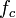$f_c\,$ and Q factor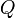$Q\,$ (i.e., damping ratio ζ) are given by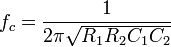$f_c = \frac{1}{ 2 \pi \sqrt{R_1R_2C_1C_2} }$

and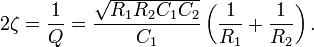$2 \zeta = \frac{1}{Q} = \frac{\sqrt{R_1R_2C_1C_2}}{C_1} \left( \frac{1}{R_1} + \frac{1}{R_2} \right).\,$

So,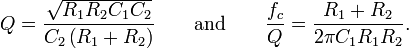$Q = \frac{ \sqrt{ R_1 R_2 C_1 C_2 } }{ C_2 \left( R_1 + R_2 \right) } \qquad \text{and} \qquad \frac{ f_c }{ Q } = \frac{R_1 + R_2}{2 \pi C_1 R_1 R_2}.\,$

The$Q\,$ factor determines the height and width of the peak of the frequency response of the filter. As this parameter increases, the filter will tend to "ring" at a single resonant frequency near$f_c\,$ (see "LC filter" for a related discussion).

A designer must choose the$Q\,$ and$f_c\,$ appropriate for his application. For example, a second-order Butterworth filter, which has maximally flat passband frequency response, has a$Q\,$ of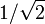$1/\sqrt{2}\,$. Because there are two parameters and four unknowns, the design procedure typically fixes one resistor as a ratio of the other resistor and one capacitor as a ratio of the other capacitor. One possibility is to set the ratio between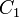$C_1\,$ and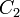$C_2\,$ as$n\,$ and the ratio between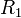$R_1\,$ and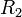$R_2\,$ as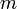$m\,$. So,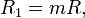$R_1=mR,\,$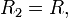$R_2=R,\,$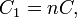$C_1=nC,\,$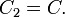$C_2=C.\,$

Therefore, the$f_c\,$ and$Q\,$ expressions are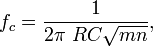$f_c = \frac{1}{2\pi\ RC\sqrt{mn}},\,$

and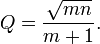$Q = \frac{\sqrt{mn}}{m+1}.$Figure 3: A low-pass filter, which is implemented with a Sallen–Key topology, with fc=15.9 kHz and Q = 0.5.

For example, the circuit in Figure 3 has an$f_c\,$ of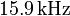$15.9\,\text{kHz}\,$ and a$Q\,$ of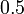$0.5\,$. The transfer function is given by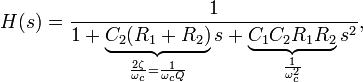$H(s)=\frac{1}{1+\underbrace{C_2(R_1+R_2)}_{\frac{2 \zeta}{\omega_c} = \frac{1}{\omega_c Q} }s+\underbrace{C_1C_2R_1R_2}_{\frac{1}{\omega_c^2}}s^2},$

and, after substitution, this expression is equal to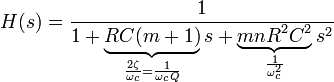$H(s)=\frac{1}{1+\underbrace{RC(m+1)}_{\frac{2 \zeta}{\omega_c} = \frac{1}{\omega_c Q} }s+\underbrace{mnR^2C^2}_{\frac{1}{\omega_c^2}}s^2}$

which shows how every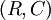$(R,C)\,$ combination comes with some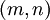$(m,n)\,$ combination to provide the same fc and Q for the low-pass filter. A similar design approach is used for the other filters below.

#### Example: High-pass filter

A second-order unity-gain high-pass filter with$f_c\,$ of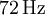$72\,\text{Hz}\,$ and$Q\,$ of$0.5\,$ is shown in Figure 4.

A second-order unity-gain high-pass filter has the transfer function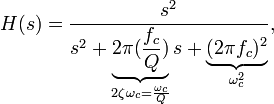$H(s) = \frac{s^2}{s^2+\underbrace{2\pi(\frac{f_c}{Q})}_{2 \zeta \omega_c = \frac{\omega_c}{Q}}s+\underbrace{(2\pi f_c)^2}_{\omega_c^2}},$

where cutoff frequency$f_c\,$ and$Q\,$ factor are discussed above in the low-pass filter discussion. The circuit above implements this transfer function by the equations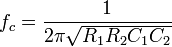$f_c = \frac{1}{2\pi\sqrt{R_1R_2C_1C_2}}\,$

(as before), and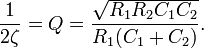$\frac{1}{2\zeta} = Q = \frac{\sqrt{R_1R_2C_1C_2}}{R_1(C_1+C_2)}.\,$

So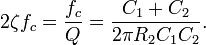$2 \zeta f_c = \frac{ f_c }{ Q } = \frac{ C_1 + C_2 }{ 2 \pi R_2 C_1 C_2 }.$

Follow an approach similar to the one used to design the low-pass filter above.

## VCVS Example: Bandpass configuration

An example of a non-unity-gain bandpass filter implemented with a VCVS filter is shown in Figure 5. Although it uses a different topology and an operational amplifier configured to provide non-unity-gain, it can be analyzed using similar methods as with the generic Sallen–Key topology. Its transfer function is given by: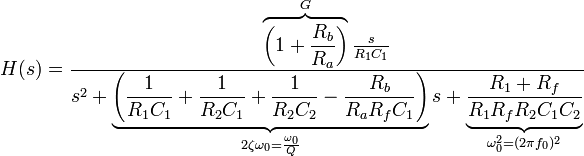$H(s) = \frac{\overbrace{\left(1+\frac{R_b}{R_a}\right)}^{G} \frac{s}{R_1 C_1}}{s^2 + \underbrace{\left( \frac{1}{R_1 C_1} + \frac{1}{R_2 C_1} + \frac{1}{R_2 C_2} - \frac{R_b}{R_a R_f C_1} \right)}_{2 \zeta \omega_0 = \frac{\omega_0}{Q}} s + \underbrace{\frac{R_1 + R_f}{R_1 R_f R_2 C_1 C_2}}_{\omega_0^2 = (2\pi f_0)^2}}$

The center frequency f0 (i.e., the frequency where the magnitude response has its peak) is given by: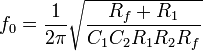$f_0=\frac{1}{2\pi}\sqrt{\frac{R_f+R_1}{C_1C_2R_1R_2R_f}}$

The voltage divider in the negative feedback loop controls the gain. The "inner gain" G provided by the operational amplifier is given by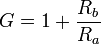$G=1+\frac{R_b}{R_a}$

while the amplifier gain at the peak frequency is given by: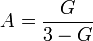$A=\frac{G}{3-G}$

It can be seen that G must be kept below 3 or else the filter will oscillate. The filter is usually optimized by selecting R2 = 2R1 and C1 = C2.